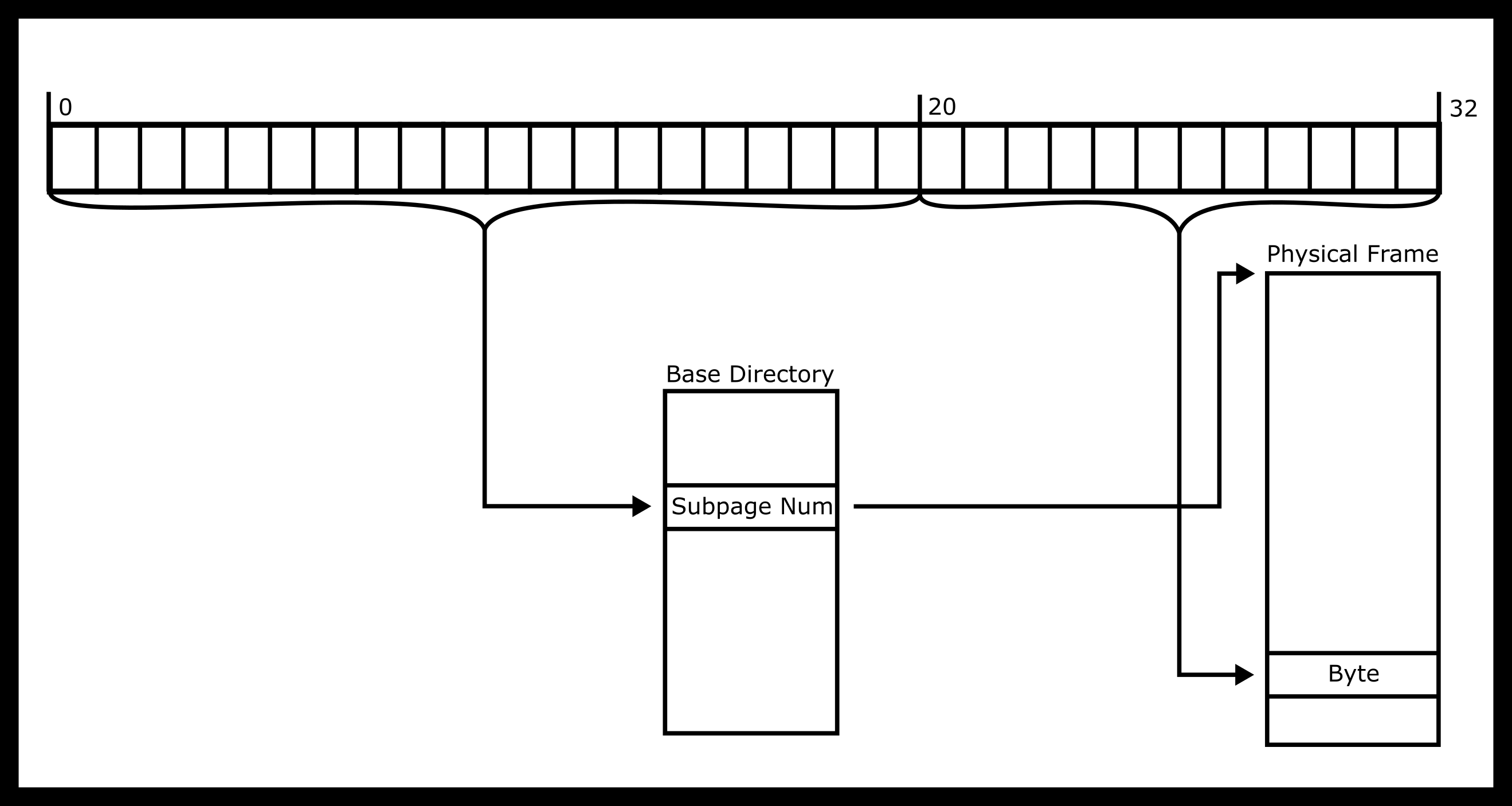# Virtual Memory

## What’s in a memory address?

Suppose I have an integer `p` allocated on the stack, and the value of `&p` is `0xAB0` (= 2736). What does this mean?

In very old computers, this would imply that `p` is stored starting at the 2736th byte in physical memory of the computer. However, with modern computers, this is no longer true. Modern operating systems and computers rely on virtual memory to better abstract the idea of computer memory.

Consider a very simple virtual memory setup. The kernel maintains a lookup table - every memory address given to a process can be looked up in this table to see where it really lies in RAM. So the kernel could do `lookup(0xAB0)` and know where to actually find some data being requested by a program. Let’s suppose the system was 32 bits, so there are a total of 2^32 possible memory addresses (1 address = 1 byte). I can fit each address in a 4 byte (32 bit) integer - but my total storage required to maintain a lookup table would be `4 bytes x 2^32 addresses` = 16 GB. This is a lot of memory!

In order to get around this issue, we can address memory as “pages”. A very common page size is 4 kilobytes. Now, I can maintain a lookup table that only refers to segments of 4 KB in RAM, instead of individual bytes. On a 32 bit system, there would be only 2^20 4 KB pages, so I can easily store a lookup table on the system. But now how do I actually access specific bytes?

The answer is to split up the memory addresses we give to the user into two segments. On a 32 bit system, we can use the first 20 bits of the address to tell us which page the byte we’re looking for is in. So we can do a lookup in the page table to find out where in physical RAM that page is actually stored. Then, the bottom 12 bits can be used to tell us the exact offset into that region of RAM to get the byte we want.

The lookup process then looks something like this:## Virtual Memory Visualization## How many pages are there in a 32bit machine (assume frame size of 4KB)?

Remember that 2^10 is 1024, so 2^20 is a bit more than one million.

For a 64 bit machine, 2^64 / 2^12 = 2^52, which is roughly 10^15 pages.

### On a 32 bit machine with 2KB pages and 4 Byte entries, how big would a single layered page table have to be to address all of the physical memory?

There are 2^32 addresses, and each page is 2^11 bytes large. So there are a total of 2^21 pages. The page directory needs to hold a 4 byte entry corresponding to each page, so the total memory uses is 4 bytes * 2^21 = 8 MB.

## Multi Leveled Page Table

In 64 bit systems, the amount of 4 KB pages is very high (10^15 from the previous slides). We can no longer afford to use a single massive page directory, so we can layer the page tables.## What is an MMU? What is a TLB.

The memory management unit in modern computing is a physical component inside a computer that is responsible for handling virtual memory requests from the CPU. In this lab, you will be implementing portions of an MMU in C.The translation look-aside buffer is a kind of “cache” for the MMU where the most recently used addresses will be stored. That way, addresses that are being accessed frequently can avoid having to be translated into physical memory, and the buffer can tell the system directly where to go in RAM to find a piece of memory.